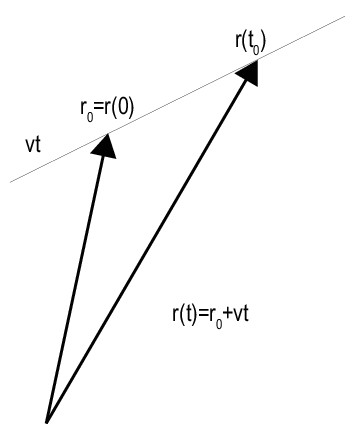Documentation

## Motion Modeling in Phased Array Systems

### Support for Motion Modeling

A critical component in phased array system applications is the ability to model motion in space. Such modeling includes the motion of arrays, targets, and sources of interference. For convenience, you can ignore the distinction between these objects and collectively model the motion of a platform.

Extended bodies can undergo both translational and rotational motion in space. Phased Array System Toolbox™ software supports modeling of translational motion.

Modeling translational platform motion requires the specification of a position and velocity vector. Specification of a position vector implies a coordinate system. In the Phased Array System Toolbox, platform position and velocity are specified in a Global Coordinate System. You can think of the platform position as the displacement vector from the global origin or as the coordinates of a point with respect to the global origin.

Let r0 denote the position vector at time 0 and v denote the velocity vector. The position vector of a platform as a function of time, r(t), is:The following figure depicts the vector interpretation of translational motion.When the platform represents a sensor element or array, it is important to know the orientation of the element or array local coordinate axes. For example, the orientation of the local coordinate axes is necessary to extract angle information from incident waveforms. See Global and Local Coordinate Systems for a description of global and local coordinate systems in the software. Finally, for platforms with varying velocity, you must be able to update the velocity vector over time.

You can model platform position, velocity, and local axes orientation with the `phased.Platform` object.

### Platform Motion with Constant Velocity

Beginning with a simple example, model the motion of a platform over ten time steps. To determine the time step, assume that you have a pulse transmitter with a pulse repetition frequency (PRF) of 1 kilohertz. Accordingly, the time interval between each pulse is 1 millisecond. Set the time step equal to pulse repetition interval.

```PRF = 1e3; Tstep = 1/PRF; Nsteps = 10;```

Next, construct a platform object specifying the platform initial position and velocity. Assume that the initial position of the platform is 100 meters from the origin at (60,80,0). Assume the speed is approximately 30 meters per second (m/s) with the constant velocity vector given by (15,25.98,0) .

`platform = phased.Platform('InitialPosition',[60;80;0],'Velocity',[15;25.98;0]);`

The orientation of the local coordinate axes of the platform is the value of the `InitialOrientationAxes` property. You can view the value of this property by entering `hplat.InitialOrientationAxes` at the MATLAB™ command prompt. Because the `InitialOrientationAxes` property is not specified in the construction of the `phased.Platform` System object™, the property is assigned its default value of `[1 0 0;0 1 0;0 0 1]`. Use the `step` method to simulate the translational motion of the platform.

```initialPos = platform.InitialPosition; for k = 1:Nsteps pos = platform(Tstep); end finalPos = pos + platform.Velocity*Tstep; distTravel = norm(finalPos - initialPos)```
```distTravel = 0.3000 ```

The `step` method returns the current position of the platform and then updates the platform position based on the time step and velocity. Equivalently, the first time you invoke the `step` method, the output is the position of the platform at t = 0.

Recall that the platform is moving with a constant velocity of approximately 30 m/s. The total time elapsed is 0.01 seconds. Invoking the `step` method returns the current position of the platform and then updates that position. Accordingly, you expect the final position to differ from the initial position by 0.30 meters. Confirm this difference by examining the value of `distTravel`.

### Platform Motion with Nonconstant Velocity

Most platforms in phased array applications do not move with constant velocity. If the time interval described by the number of time steps is small with respect to the platform speed, you can often approximate the velocity as constant. However, there are situations where you must update the platform velocity over time. You can do so with the `phased.Platform` System object� because the `Velocity` property is tunable.

This example models a target initially at rest. The initial velocity vector is (0,0,0) . Assume the time step is 1 millisecond. After 500 milliseconds, the platform begins to move with a speed of approximately 10 m/s. The velocity vector is (7.07,7.07,0). The platform continues at this velocity for an additional 500 milliseconds.

```Tstep = 1e-3; Nsteps = 1/Tstep; platform = phased.Platform('InitialPosition',[100;100;0]); for k = 1:Nsteps/2 [tgtpos,tgtvel] = platform(Tstep); end platform.Velocity = [7.07; 7.07; 0]; for k = Nsteps/2+1:Nsteps [tgtpos,tgtvel] = platform(Tstep); end```

### Track Range and Angle Changes Between Platforms

This example uses the `phased.Platform` System object™ to model the change in range between a stationary radar and a moving target. The radar is located at (1000,1000,0) and has a velocity of (0,0,0) . The target has an initial position of (5000,8000,0 ) and moves with a constant velocity of (?30,?45,0). The pulse repetition frequency (PRF) is 1 kHz. Assume that the radar emits ten pulses.

```PRF = 1e3; Tstep = 1/PRF; radar = phased.Platform('InitialPosition',[1000;1000;0]); target = phased.Platform('InitialPosition',[5000;8000;0],... 'Velocity',[-30;-45;0]);```

Calculate initial target range and angle.

`[initRng, initAng] = rangeangle(target.InitialPosition,radar.InitialPosition);`

```v = radialspeed(target.InitialPosition,target.Velocity,... radar.InitialPosition);```

Simulate target motion.

```Npulses = 10; for num = 1:Npulses tgtpos = target(Tstep); end```

Compute last target position.

`tgtpos = tgtpos + target.Velocity*Tstep;`

Calculate final target range and angle.

```[finalRng,finalAng] = rangeangle(tgtpos,radar.InitialPosition); deltaRng = finalRng - initRng```
```deltaRng = -0.5396 ```

The constant velocity of the target is approximately 54 m/s. The total time elapsed is 0.01 seconds. The range between the target and the radar should decrease by approximately 54 centimeters. Compare the initial range of the target, `initRng`, to the final range, `finalRng`, to confirm that this decrease occurs.